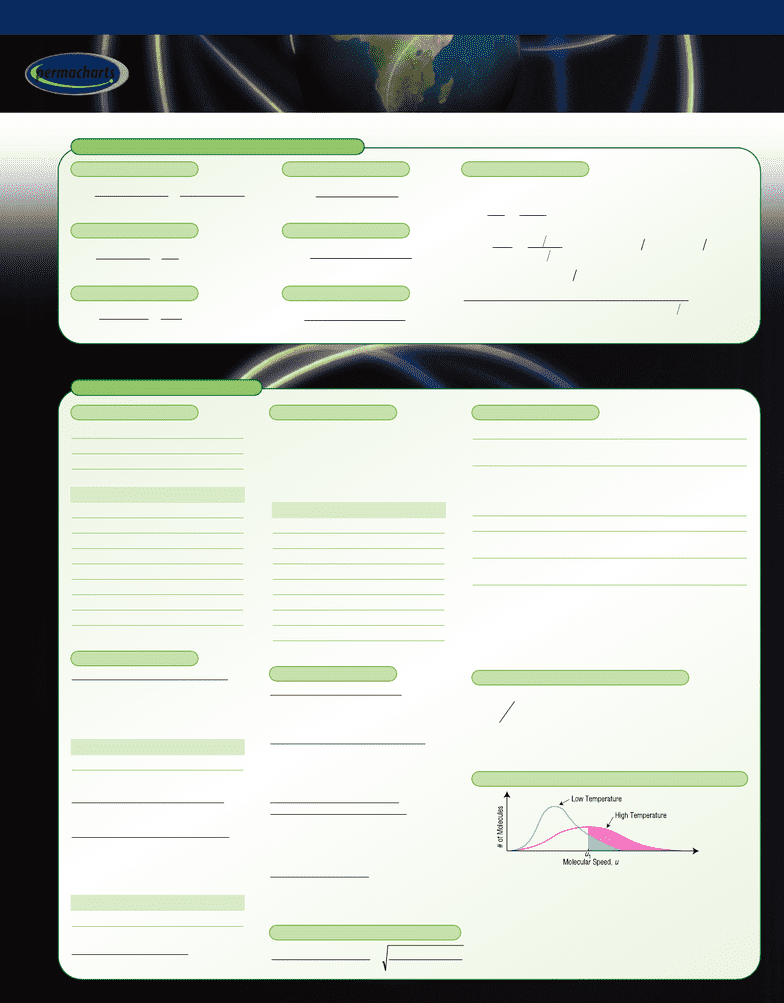Get 2 days of unlimited access
Class Notes (1,000,000)
US (420,000)
Chicago (800)
CHEM (30)
All (4)
Reference Guide

# Introductory Chemistry - Reference Guides

Department
Chemistry
Course Code
CHEM 11100
Professor
All
Chapter
Permachart

This preview shows page 1. to view the full 4 pages of the document.MASS, MOLES, & CONCENTRATIONS
THE GASEOUS STATE
Introductory Chemistry
Introductory Chemistry
1ppm = 10 mass percent = 10 mass fraction
= where:
= where:
Molarity Liters solution Total moles
Molarity
Mass fraction of solvent Density of solution kg L
46
Xn
n
nM
nM mnM m nM m
mm
m
mM
mM XmM n mM n
X
m
AA
i
AA
ii
fAAA A ii i
fAA
i
AA
ii
AAA A ii i
A
===
===
×=
×
()
=
,
,
nm
M
AA
A
==
×
Mass,
Molar mass,
# of particles
6.022 10
M
23
Xn
n
AA
i
==
=
10
Moles of A
Total moles
mm
m
fAA
i
==
10
M
Mass of A
Total mass
ppm =
10
M
Mass of substance
Total mass
106
M
[]
=
10
M
Moles dissolved solute
Liters solution
m=
10
M
Moles dissolved solute
Kilograms solvent
2
Pan VVnb nRT+
()
=
2
2
Rate of Transport of 1
Rate of Transport of 2
Molar Mass of 2
Molar Mass of 1
=
{
pressure correction term
a= measure of attractive force
b= measure of repulsive force or excluded volume
{
volume correction term
• As temperature increases, the average molecular speed
increases, causing the curve to shift to the right and
NUMBER OF MOLES OF A
MOLE FRACTION OF A
MASS FRACTION OF A
PARTS PER MILLION
MOLARITY
MOLALITY
CONVERSIONS
TEMPERATURE
°C (Celsius) = 5/9(°F – 32)
°F (Fahrenheit) = (9/5)°C + 32
K (Kelvin) = °C + 273.15
°R (Rankin) = °F + 459.67
PRESSURE
1 atm = 101325 Pa
= 1.01325 bar
= 14.6959 psi
= 760 mm Hg
= 760 Torr
GAS MIXTURES
Dalton’s Law of Partial Pressures
• Partial pressure, pi, = pressure
exerted by individual component
at Tand Vof mixture
Âpi = Ptotal (T, V are constant)
SINGLE GASES
Boyle’s Law PV = Constant (at constant T, n)
Law of Charles & V/T = Constant
Gay-Lussac (at constant P, n)
Avogadro’s At constant Tand P, equal
Hypothesis volumes of different gases contain
the same number of particles
V/n = Constant (at constant T, P)
Ideal Gas Law PV = nRT (at low P, high T)
Molar Volume VMolar = 22.414 L at STP
of an Ideal Gas (STP = 0ºC, 1 atm)
Density of an d= PM/RT
Ideal Gas
Gas Constant, RR= 0.08206 L•atm/mol•K
= 82.0578 cm3•atm/mol•K
= 8.3145 J/mol•K
= 8.3145 L•kPa/mol•K
= 1.9859 cal(I.T.)/mol•K
= 0.73024 ft3•atm/lb-mol•°R
Average Molar Mass of Mixture
Mavg = niMi/ntot
Density of Ideal Mixture
dmixture = PMavg/RT
Amagat’s Law of Partial Volumes
• Partial volume, Vi, = volume
occupied by individual component
at Tand Pof mixture
ÂVi=Vtotal (T, P are constant)
MOLECULAR PROPERTIES
Average Molecular Velocity
u= (3RT/M)1/2
uN2(25°C) @500 m/s
# of Impacts per Second on 1 m2
C= 1/4(N/V)u(N = # of molecules)
CN2@1028 collisions/m2•s
(at 1 atm, 25°C)
Average Distance Between
Molecules = Mean Free Path
l = [πD2m•(N/V)]–1
Dm= diameter of molecule
lN2(1 atm) @10–7 m
Diffusion Coefficient
D= 1/3ul
DN2(1 atm, 25°C) @0.17 cm2/s
VAN DER WAALS EQUATION FOR REAL GASES
GRAHAMS LAW OF DIFFUSION/EFFUSION
MAXWELL’S VELOCITY DISTRIBUTION OF GASEOUS MOLECULES
Examples (K)
108– H-Bomb
2000 Bunsen burner (CH4/O2)
1000 – Cigarette
373 Boiling point of H2O
310 Body temperature
273 Freezing point of H2O
90 Boiling point of O2
4.2 Boiling point of He
0–Absolute zero
Examples (atm)
107 Center of sun
106 Center of earth
500–1000 – NH3synthesis
150 Gas cylinder
50–75 – CH4pipeline
1–Atmosphere
10–6 Vapor pressure of Hg
10–12 Laboratory vacuum
100 molecules/cm3(outer space)
Gas 1 Gas 2 Gas 1 & 2
1 L 1 L 1 L
T, P1T, P2T, PTotal
Gas 1 Gas 2 Gas 1 & 2
1 L 0.8 L 1.8 L
T, P T, P T, P
INTRODUCTORY CHEMISTRY • A-804-41
l e a r n r e f e r e n c e r e v i e w
TM
permacharts
© 1995-2012 Mindsource Technologies Inc.
www.permacharts.com
###### You're Reading a Preview

Unlock to view full version